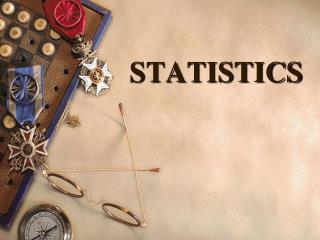# STATISTICS - PowerPoint PPT PresentationDownload PresentationSTATISTICS

STATISTICSDownload Presentation## STATISTICS

- - - - - - - - - - - - - - - - - - - - - - - - - - - E N D - - - - - - - - - - - - - - - - - - - - - - - - - - -
##### Presentation Transcript

1. STATISTICS

2. CONTENTS OF THE CHAPTER 1. Mean By direct method By assumed mean method By step-deviation method 2. Median Of grouped data Of ungrouped data 3.Mode Of grouped data Of ungrouped data

3. 4. Graphical representation of cumulative frequency distribution Less than type cumulative frequency distribution More than type frequency distribution Also finding the missing frequencies when Mean is given, when Median is given etc.

4. Mean: Mean is the number which is obtained by adding the values of all the items of a series and dividing the total by the number of items. Median:According to CANNOR, “The median is that value of the variable which divides the group into two equal parts,one part comprising all values greater than the median and the other part comprising all the values smaller than the median value.” 3) Mode: According to KENNY, “The value of the variable which occurs most frequently in a distribution is called the mode.”

5. Mean of raw data If xı, x2, xз------------------------xn are n values of a variable X, then the arithmetic mean of these values is defined as: Mean= x1+x2+x3------------+xn / n In other words, we can say that the Arithmetic mean of a set of observations is equal to their sum divided by total number of observations.

6. Example: The first 10 students of class 10TH A scored the following marks in 2nd unit test. 35,30,32,40,35,40,25,20,25,30 Find the mean. Here x1=35,x2=30, x3=32--------------x10=30 Mean=x1+x2+x3-----------+x10 / 10 Mean=35+30+32+40+35+40+25+20+25+30 / 10 =312 / 10 =31.2 Average= mean marks= 31.2

7. In most of our real life situations, data is usually so large that to make a meaningful study it needs to be condensed as grouped data. So, we need to convert given ungrouped data into grouped data and devise same method to find its mean.

8. Example: The marks obtained by 30 students of class 10TH of a certain school in mathematics paper consisting of 100 marks are presented below. Find out the mean of the marks obtained by the students. Marks obtained(x1) no. of students(f1) 10 1 20 1 36 3 40 4 50 3 56 2 60 4 70 4 72 1 80 1

9. 88 2 92 3 95 1 Solution : To find mean marks, we require the product of each x1 with the corresponding frequency f1. So, let us put them in a column as shown:

10. Marks(x1) No. of stds(f1) f1x1 10 1 10 20 1 20 36 3 108 40 4 160 50 3 150 56 2 112 60 4 240 70 4 280 72 1 72 80 1 80 88 2 176 92 3 276 95 1 95 Σf1=30 Σf1x1=1779

11. Now Mean= Σf1x1 = 1779 = 59.3 Σf1 30 therefore, the mean marks obtained is 59.3.

12. The following algorithm may be used to compute Arithmetic mean by DIRECT METHOD: • Prepare the frequency table. • Multiply the frequency of each row with the corresponding values of variable to obtain third column containing f1x1. • Find the sum of all entries in column 3 to obtain Σf1x1. • Find the sum of all the frequencies in column 2 to obtain Σf1= N. • Use the formula Mean= Σf1x1 N

13. Now let us convert the data taken in the previous example by forming class-intervals of width say 15. Remember that, while calculating frequencies to each class-interval, students falling in any upper class-limit would be considered in the next class. Example: 4 students who have obtained 40 marks would be considered in the class-interval of 40-55 and not in 25-40. With this convention in our mind, let us form a grouped frequency distribution table as under

14. Class interval no. of students 10-25 2 25-40 3 40-55 7 55-70 6 70-85 6 85-100 6 Now for each class-interval, we require a point which would serve as the representative of the whole class. It is assumed that the frequency of each class-interval is centered around its mid-points.

15. So,we find the mid-point of a class(or its class mark) by finding the average of its upper and lower limits. That is class mark= upper limit+lower limit 2 i.e. for the class 10-25, the class mark is 10+25 =17.5 2 Similarly we can find class marks of the remaining class-intervals. These class marks serve as our x1. Now we can proceed to compute the mean in the same manner.

16. class-interval no. of stds class mark f1x1 (f1) (x1) 10-25 2 17.5 35.0 25-40 3 32.5 97.5 40-55 7 47.5 332.5 55-70 6 62.5 375.0 70-85 6 77.5 465.0 85-100 6 92.5 555.0 Σf1=30 Σf1x1=1860 Mean =Σf1x1 = 1860 = 62. Σf1 30

17. We observed that of the example 2 & 3 are using the same data and employing the same formula for the calculation of the mean but the result obtained are different. Can you think WHY THIS IS SO and WHICH ONE IS MORE ACCURATE? The difference in the two values is because of the mid-point assumption in the example 3. 59.3 being the exact mean, while 62 an approximate mean.